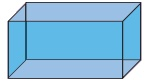Home | | Maths 8th Std | Exercise 2.3 (Three dimensional (3-D) shapes)

# Exercise 2.3 (Three dimensional (3-D) shapes)

8th Maths : Chapter 2 : Measurements: Three dimensional (3-D) shapes : Exercise 2.3: Text Book Back Exercises Questions with Answers, Solution

Exercise 2.3

1. Fill in the blanks:

(i) The three dimensions of a cuboid are ____, _____ and ____. [Answer: length, breadth, height]

(ii) The meeting point of more than two edges in a polyhedron is called as ______. [Answer: vertex]

(iii) A cube has __________ faces.  [Answer: six]

(iv) The cross section of a solid cylinder is __________. [Answer: circle]

(v) If a net of a 3-D shape has six plane squares, then it is called ______. [Answer: cube]

2. Match the following:3. Which 3-D shapes do the following nets represent? Draw themSolution:

(i) The net represents cube, because it has 6 squares.(ii) The net represents cuboid(iii) The net represents Triangular prism(iv) The net represents square pyramid(v) The net represents cylinder4. For each solid, three views are given. Identify for each solid, the corresponding Top, Front and Side (T, F and S) views.Solution:5. Verify Euler’s formula for the table given below.Solution:

Euler’s formula is given by F + V − E = 2

(i) F = 4; V = 4; E = 6

F + V − E = 4 + 4 − 6 = 8 − 6

F + V − E = 2

Euler’s formula is satisfied.

(ii) F = 10; V = 6; E = 12

F + V − E = 10 + 6 – 12 = 16 − 12 = 4 ≠ 2

Euler’s formula is not satisfied.

(iii) F = 12 ; V = 20 ; E = 30

F + V − E = 12 + 20 − 30 = 32 − 30 = 2

∴  Euler’s formula is satisfied.

(iv) F = 20 ; V = 13 ; E = 30

F + V − E = 20 + 13 − 30 = 33 − 30 = 3 ≠ 2

∴  Euler’s formula is not satisfied.

(v) F = 32 ; V = 60 ; E = 90

F + V − E = 32 + 60 − 90 = 92 − 90 = 2

∴  Euler’s formula is satisfied.

Exercise 2.3

1. (i) length, breadth and height (ii) Vertex (iii) Six (iv) Circle (v) Cube

2. (i) (b) (ii) (a) (iii) (d) (iv) (c)

3. (i) Cube (ii) Cuboid (iii) Triangular Prism (iv) Square Pyramid (v) Cylinder

4. (i) F, T, S (ii) T,S,F (iii) S,F,T

5. (i) Yes (ii) No (iii) Yes (iv) No (v) Yes

Tags : Questions with Answers, Solution | Measurements | Chapter 2 | 8th Maths , 8th Maths : Chapter 2 : Measurements
Study Material, Lecturing Notes, Assignment, Reference, Wiki description explanation, brief detail
8th Maths : Chapter 2 : Measurements : Exercise 2.3 (Three dimensional (3-D) shapes) | Questions with Answers, Solution | Measurements | Chapter 2 | 8th Maths